# 【Attention Transfer】paying more attention to attention¶

## 介绍¶

• 非注意力视觉过程可以用于感知整体场景，聚合高层信息，可以用于控制注意过程，引导到场景的某个部分。

• 但是不同的视觉系统的观察角度可能不相同，如何利用注意力信息来提升性能是一个值得研究的问题。

• 更具体来讲如何使用教师网络来提升其他学生网络的性能。

• activation-based spatial attention maps

• 提出使用attention作为迁移知识的特殊机制。

• 提出同时使用activation-based 和 gradient-based spatial attention maps

• 实验证明可以有一定的提升，并且要比full-activation transfer性能更好，可以与KD相结合。

## Attention Transfer¶

### 1. Activation-based Attention Transfer¶

\mathcal{F}: R^{C \times H \times W} \rightarrow R^{H \times W}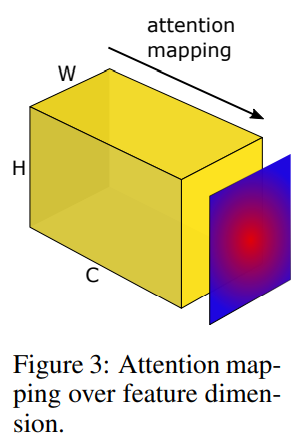• 求和：$F_{\mathrm{sum}}(A)=\sum_{i=1}^{C}\left|A_{i}\right|$

• 求P范数（p>1）：$F_{\text {sum }}^{p}(A)=\sum_{i=1}^{C}\left|A_{i}\right|^{p}$

• 求max P范数（p>1）: $F_{\max }^{p}(A)=\max _{i=1, C}\left|A_{i}\right|^{p}$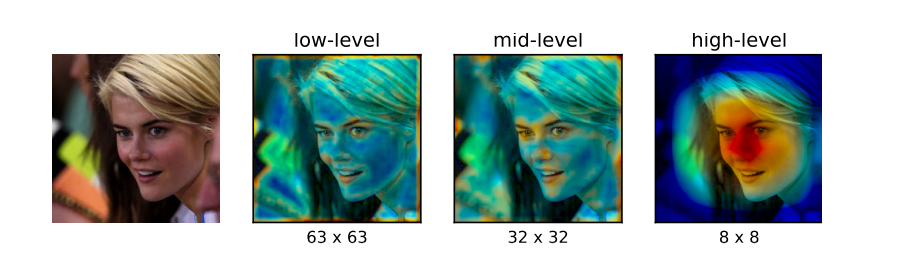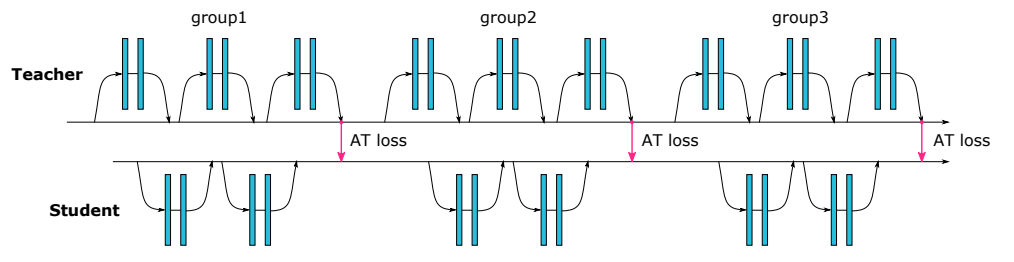\mathcal{L}_{A T}=\mathcal{L}\left(\mathbf{W}_{S}, x\right)+\frac{\beta}{2} \sum_{j \in \mathcal{I}}\left\|\frac{Q_{S}^{j}}{\left\|Q_{S}^{j}\right\|_{2}}-\frac{Q_{T}^{j}}{\left\|Q_{T}^{j}\right\|_{2}}\right\|_{p}

L(ws,x)是交叉熵公式，Q代表对应层的attention maps，第二项使用的是L2范数。

ps：如果空间特征图尺寸不匹配，会采用插值的方式保持一致。所以这启发我们可以使用通道注意力，虽然可解释性没那么强，但是扩展性好。

J_{S}=\frac{\partial}{\partial x} \mathcal{L}\left(\mathbf{W}_{\mathbf{S}}, x\right), J_{T}=\frac{\partial}{\partial x} \mathcal{L}\left(\mathbf{W}_{\mathbf{T}}, x\right)

\mathcal{L}_{A T}\left(\mathbf{W}_{\mathbf{S}}, \mathbf{W}_{\mathbf{T}}, x\right)=\mathcal{L}\left(\mathbf{W}_{\mathbf{S}}, x\right)+\frac{\beta}{2}\left\|J_{S}-J_{T}\right\|_{2}

\frac{\partial}{\partial \mathbf{W}_{\mathbf{S}}} \mathcal{L}_{A T}=\frac{\partial}{\partial \mathbf{W}_{\mathbf{S}}} \mathcal{L}\left(\mathbf{W}_{\mathbf{S}}, x\right)+\beta\left(J_{S}-J_{T}\right) \frac{\partial^{2}}{\partial \mathbf{W}_{\mathbf{S}} \partial x} \mathcal{L}\left(\mathbf{W}_{\mathbf{S}}, x\right)

## 实验部分¶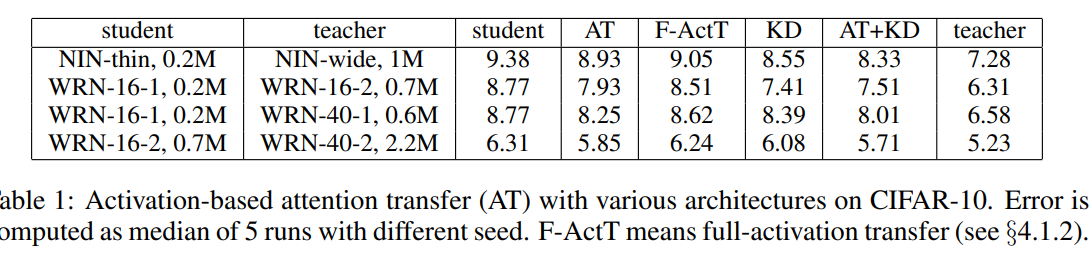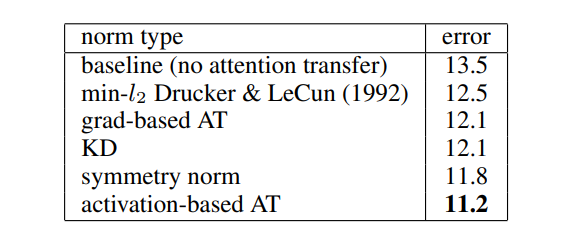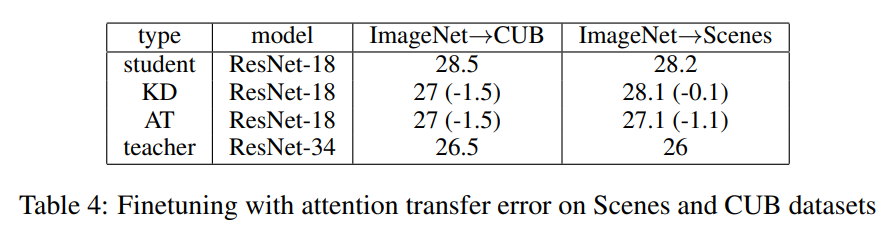## 实现¶

  def f(input, params, mode, base=''):
g0 = group(x, params, f'{base}group0', mode, 1)
g1 = group(g0, params, f'{base}group1', mode, 2)
g2 = group(g1, params, f'{base}group2', mode, 2)
o = F.relu(utils.batch_norm(g2, params, f'{base}bn', mode))
o = F.avg_pool2d(o, 8, 1, 0)
o = o.view(o.size(0), -1)
o = F.linear(o, params[f'{base}fc.weight'], params[f'{base}fc.bias'])
return o, (g0, g1, g2)


KD hinton实现：

def distillation(y, teacher_scores, labels, T, alpha):
p = F.log_softmax(y/T, dim=1)
q = F.softmax(teacher_scores/T, dim=1)
l_kl = F.kl_div(p, q, size_average=False) * (T**2) / y.shape
l_ce = F.cross_entropy(y, labels)
return l_kl * alpha + l_ce * (1. - alpha)


feature map l2 loss实现：

def at_loss(x, y):
return (at(x) - at(y)).pow(2).mean()


y_s, y_t, loss_groups = utils.data_parallel(f, inputs, params, sample, range(opt.ngpu))
loss_groups = [v.sum() for v in loss_groups]
return utils.distillation(y_s, y_t, targets, opt.temperature, opt.alpha) + opt.beta * sum(loss_groups), y_s